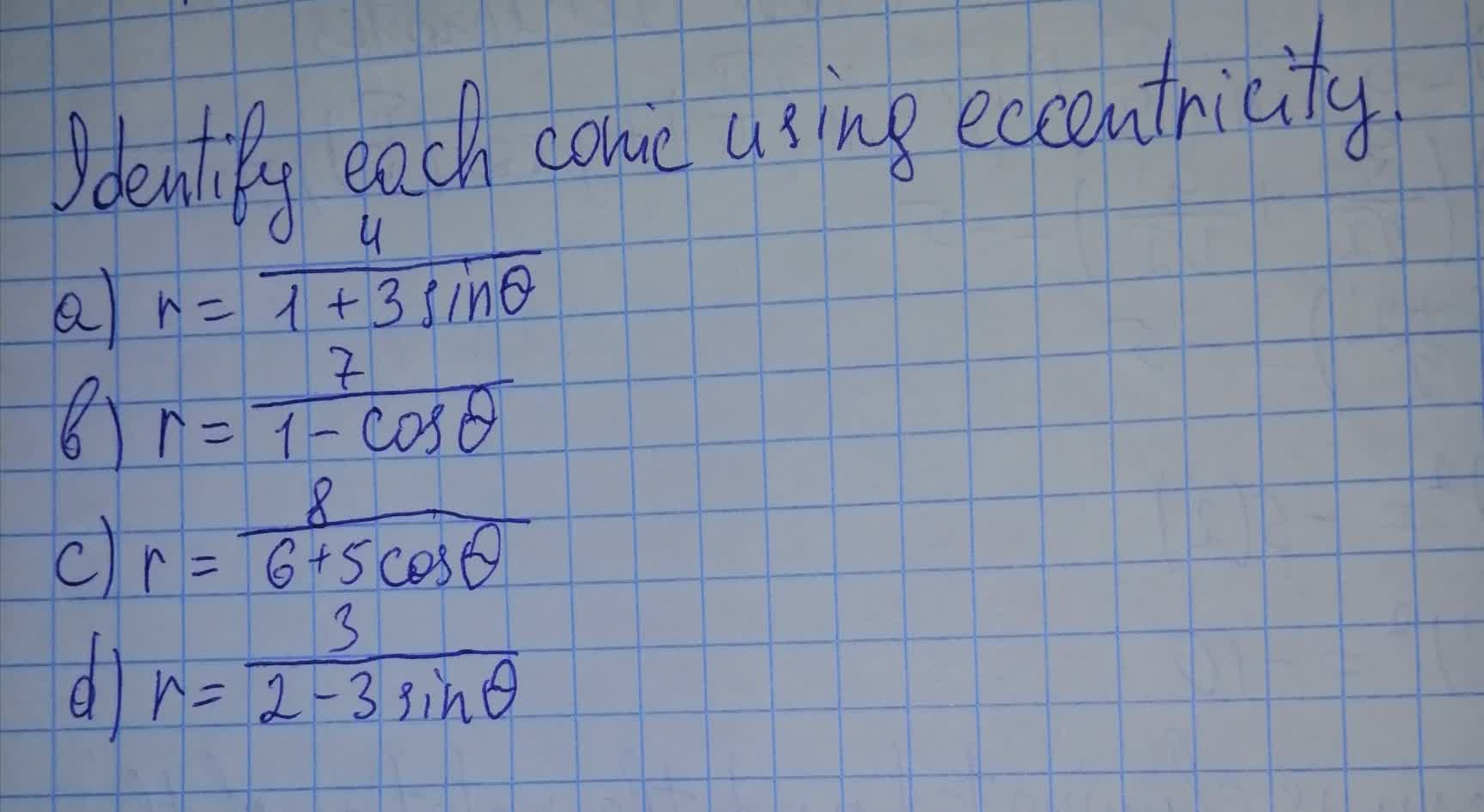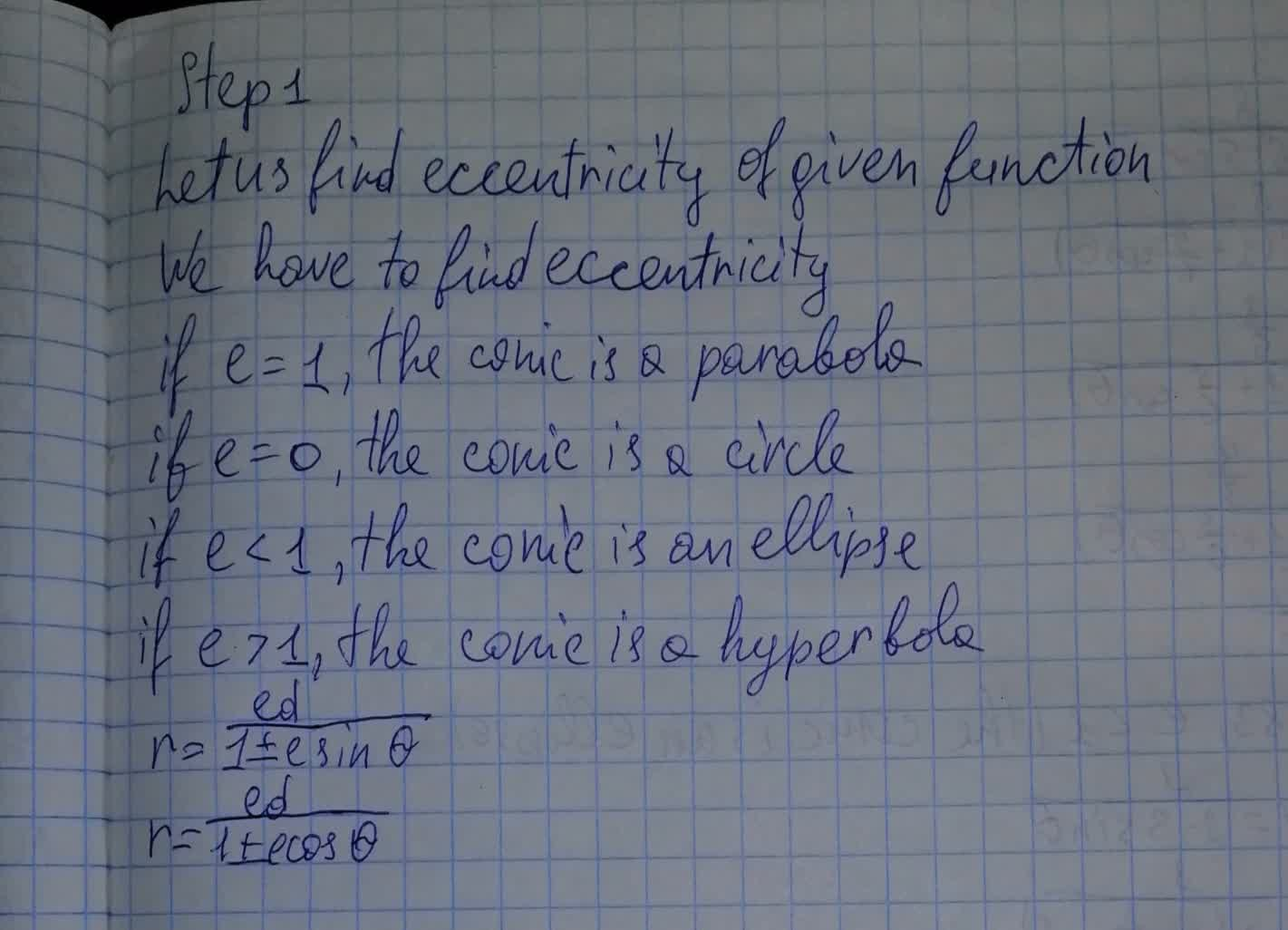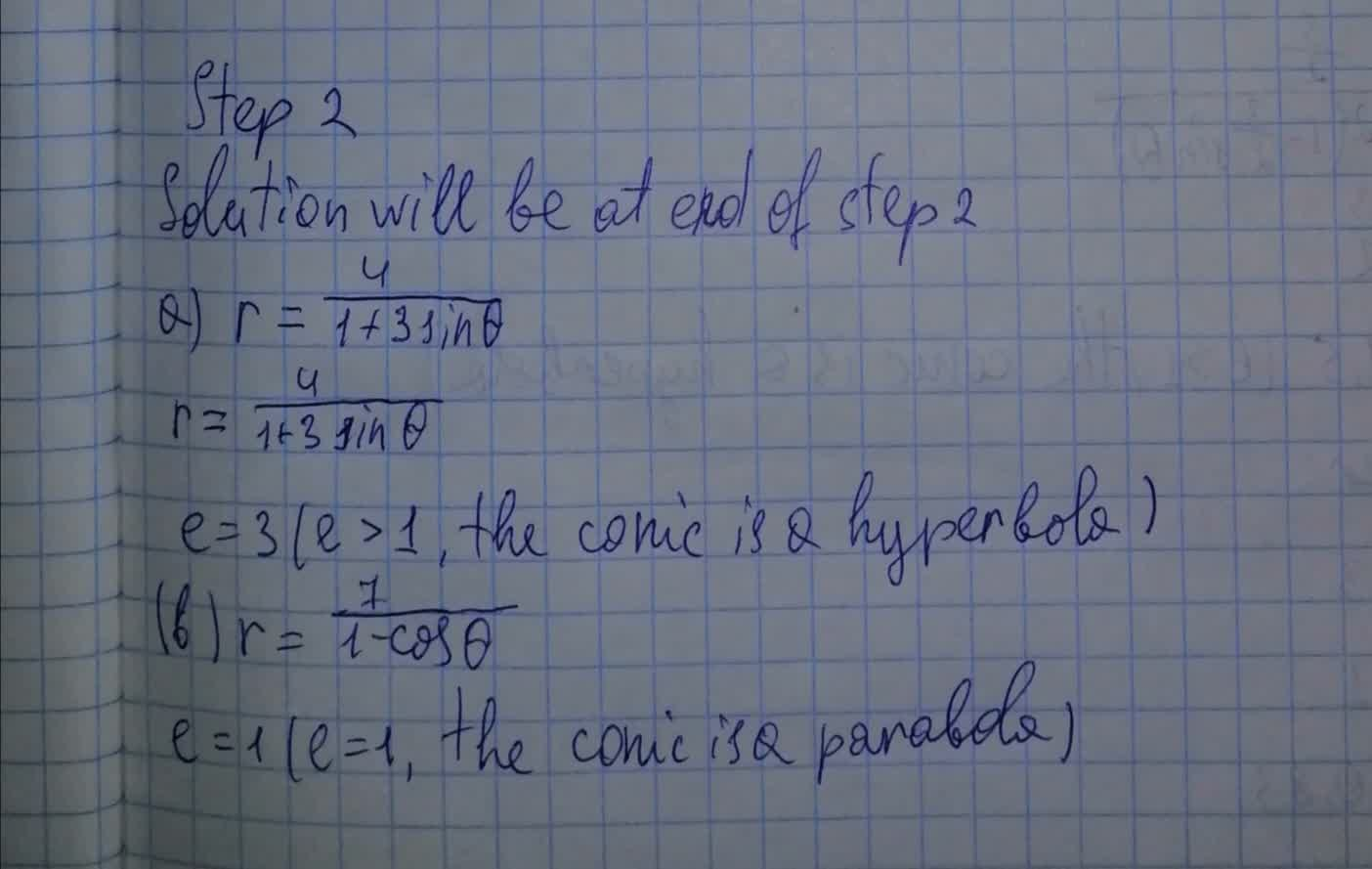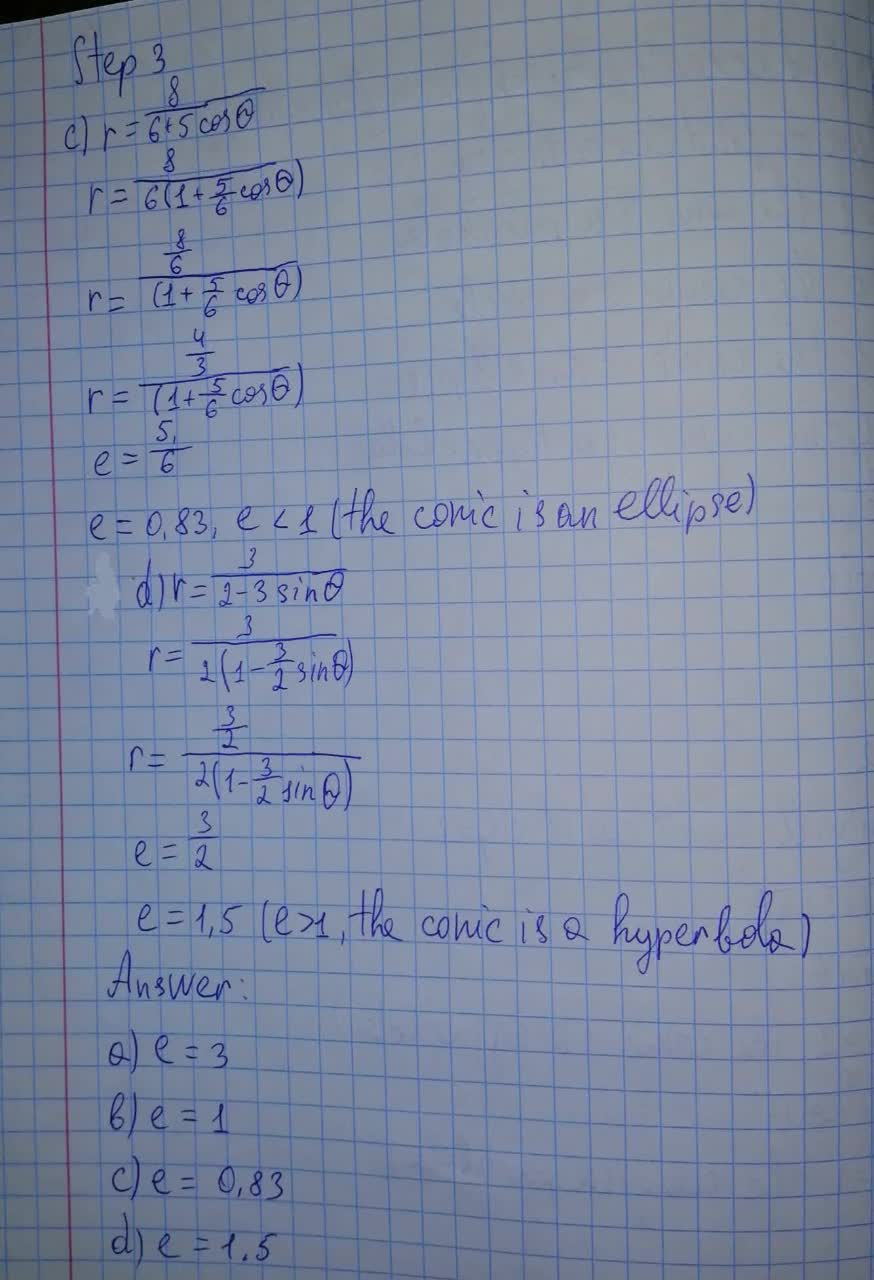# Identify (a) r=\frac{4}{1+3\sin \theta} (b) r=\frac{7}{1-3\cos \theta} (c) r=\frac{8}{6+5\cos \theta} (d) r=\frac{3}{2-3\sin \theta}Bevan Mcdonald 2021-08-14 Answered

Identify each conic using eccentricity.
(a) $r=\frac{4}{1+3\mathrm{sin}\theta }$
(b) $r=\frac{7}{1-3\mathrm{cos}\theta }$
(c) $r=\frac{8}{6+5\mathrm{cos}\theta }$
(d) $r=\frac{3}{2-3\mathrm{sin}\theta }$You can still ask an expert for help

• Questions are typically answered in as fast as 30 minutes

Solve your problem for the price of one coffee

• Math expert for every subject
• Pay only if we can solve itEzra Herbert

Identifying:###### Not exactly what you’re looking for?Vasquez

Step 1

Let us find eccentricity of given function

We have to find eccentricity

if e=1, the conic is a parabola

if e=0, the conic is a circle

if e<1, the conic us an ellipse

if e>1, the conic is a hyperbola

$r=\frac{ed}{1±e\mathrm{sin}\theta }$

$r=\frac{ed}{1±e\mathrm{cos}\theta }$

Step 2

Solution will be at end of step 2

a)$r=\frac{4}{1+3\mathrm{sin}\theta }$

$r=\frac{4}{1+3\mathrm{sin}\theta }$

e=3 (e>1, the conic is a hyperbola)

b) $r=\frac{7}{1-\mathrm{cos}\theta }$

e=1(e=1, the conic is a parabola)

Step 3

c) $r=\frac{8}{6+5\mathrm{cos}\theta }$

$r=\frac{8}{6\left(1+\frac{5}{6}\mathrm{cos}\theta \right)}$

$r=\frac{\frac{8}{6}}{\left(1+\frac{5}{6}\mathrm{cos}\theta \right)}$

$r=\frac{\frac{4}{3}}{\left(1+\frac{5}{6}\mathrm{cos}\theta \right)}$

$e=\frac{5}{6}$

e=0.83, e<1 (the conic is an ellipse)

d) $r=\frac{3}{2-3\mathrm{sin}\theta }$

$r=\frac{3}{2\left(1-\frac{3}{2}\mathrm{sin}\theta \right)}$

$r=\frac{\frac{3}{2}}{2\left(1-\frac{3}{2}\mathrm{sin}\theta \right)}$

$e=\frac{3}{2}$

e=1.5 (e>1, the conic is a hyperbola)

a) e=3

b) e=1

c) e=0.83

d) e=1.5